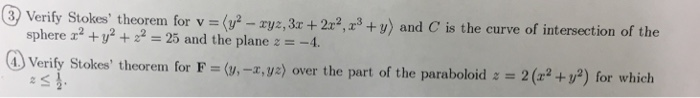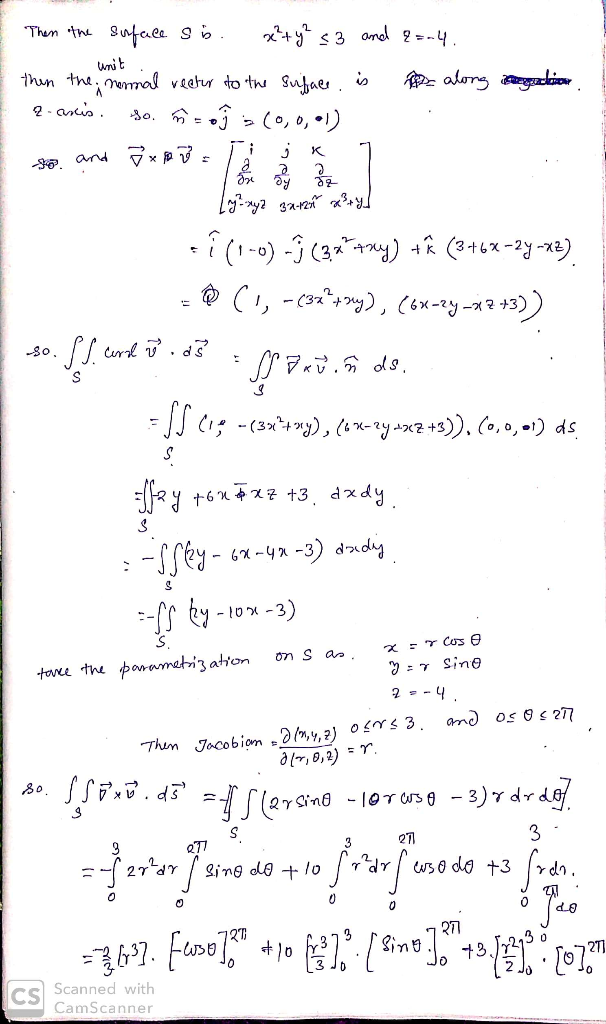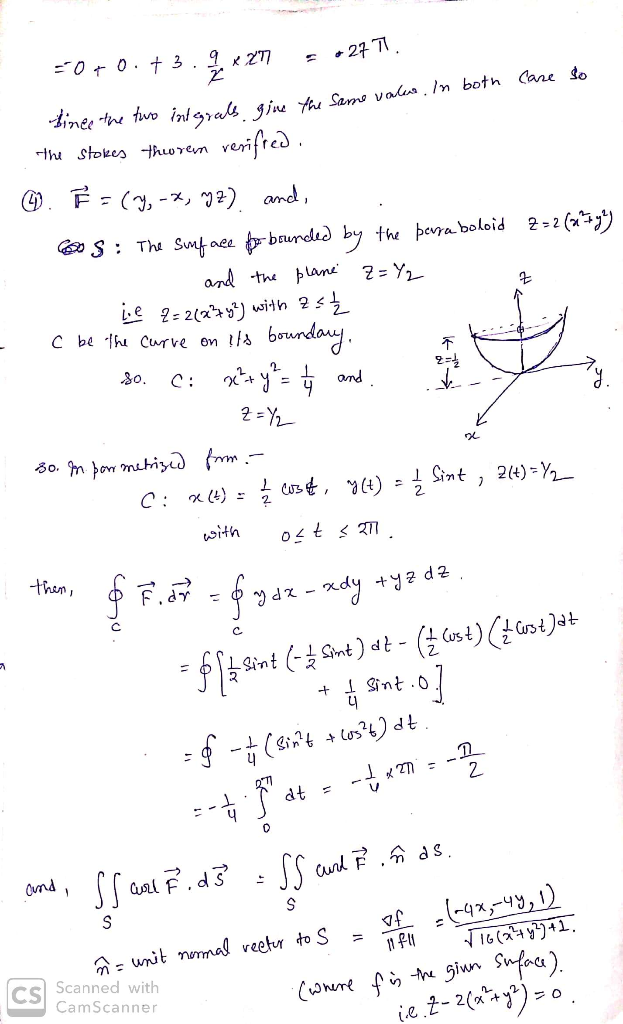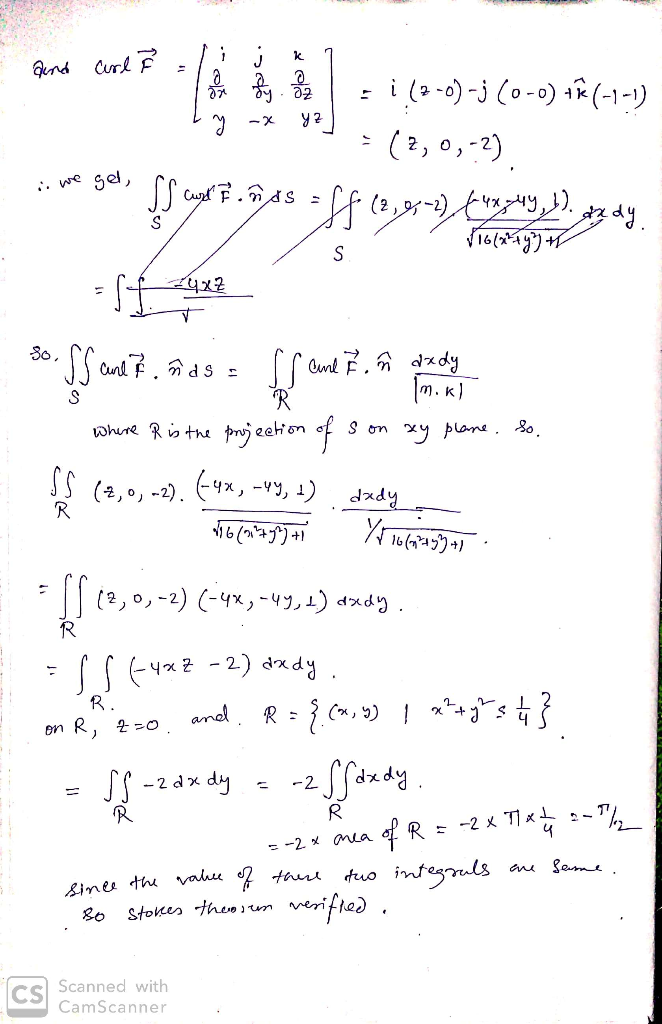Homework Help Question & Answers

# 3 Verify Stok sa theorem, for v = ys_ yz,3r+ 2x2, x3 +v) and C's the curve of intersection of the...solve using direct method then prove using Stokes’ theorem
3 Verify Stok sa theorem, for v = ys_ yz,3r+ 2x2, x3 +v) and C's the curve of intersection of the ,+y and C is the curve of intersection of the sphere x2 + y2 + 22-25 and the plane 2--4 1) Verify Stokes, theore G Verifty Stokes' theorem for F (u-x.v) over the part of the paraboloid: 2(a2+1') for which##### Add Answer of: 3 Verify Stok sa theorem, for v = ys_ yz,3r+ 2x2, x3 +v) and C's the curve of intersection of the...
More Homework Help Questions Additional questions in this topic.

• #### C is the curve of intersection of the paraboloid z (++y and the plane z 2x+2. 2. Evaluate [ F -dr using Stokes' Theorem. Choose the simplest surface with boundary curve C and orient it upward....

Need Online Homework Help?

Get FREE EXPERT Answers
WITHIN MINUTES
Related Questions